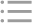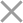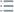## Fill out the form in 2 simple steps below:

Step 1

Step 2
** By clicking this button you agree to our Privacy Policy statementRequest our prices
--Select currency--
USD
EUR
* By clicking this button you agree to our Privacy Policy statementFree PVS‑Studio license for Microsoft MVP specialists
* By clicking this button you agree to our Privacy Policy statementTo get the licence for your open-source project, please fill out this form
* By clicking this button you agree to our Privacy Policy statementI am interested to try it on the platforms:
 Windows Linux macOS PVS-Studio for .NET Core JetBrains Rider
* By clicking this button you agree to our Privacy Policy statementMessage submitted.

Your message has been sent. We will email you at

check your Spam/Junk folder and click the "Not Spam" button for our message.
This way, you won't miss messages from our team in the future.

>
>
>
V547. Expression is always true/false.Introduction
Analyzing projects
On Windows
On Linux and macOS
Cross-platform
IDE
Build systems
Game Engines
Continuous use of the analyzer in software development
Deploying the analyzer in cloud Continuous Integration services
Managing analysis results
Analyzer diagnostics
General Analysis (C++)
General Analysis (C#)
General Analysis (Java)
Micro-Optimizations (C++)
Micro-Optimizations (C#)
Diagnosis of 64-bit errors (Viva64, C++)
Customer specific requests (C++)
MISRA errors
AUTOSAR errors
OWASP errors (C++)
OWASP errors (C#)
OWASP errors (Java)
Problems related to code analyzerContents

# V547. Expression is always true/false.

Mar 12 2012

The analyzer detected a potential error: a condition is always true or false. Such conditions do not always signal an error but still you must review such code fragments.

Consider a code sample:

``````LRESULT CALLBACK GridProc(HWND hWnd,
UINT message, WPARAM wParam, LPARAM lParam)
{
...
if (wParam<0)
{
BGHS[SelfIndex].rows = 0;
}
else
{
BGHS[SelfIndex].rows = MAX_ROWS;
}
...
}``````

The "BGHS[SelfIndex].rows = 0;" branch here will never be executed because the wParam variable has an unsigned type WPARAM which is defined as "typedef UINT_PTR WPARAM".

Either this code contains a logical error or we may reduce it to one line: "BGHS[SelfIndex].rows = MAX_ROWS;".

Now let's examine a code sample which is correct yet potentially dangerous and contains a meaningless comparison:

``````unsigned int a = _ttoi(LPCTSTR(str1));
if((0 > a) || (a > 255))
{
return(FALSE);
}``````

The programmer wanted to implement the following algorithm.

1) Convert a string into a number.

2) If the number lies outside the range [0..255], return FALSE.

The error here is in using the 'unsigned' type. If the _ttoi function returns a negative value, it will turn into a large positive value. For instance, value "-3" will become 4294967293. The comparison '0 > a' will always evaluate to false. The program works correctly only because the range of values [0..255] is checked by the 'a > 255' condition.

The analyzer will generate the following warning for this code fragment: "V547 Expression '0 > a' is always false. Unsigned type value is never < 0."

We should correct this fragment this way:

``````int a = _ttoi(LPCTSTR(str1));
if((0 > a) || (a > 255))
{
return(FALSE);
}``````

Let's consider one special case. The analyzer generates the warning:

V547 Expression 's == "Abcd"' is always false. To compare strings you should use strcmp() function.

for this code:

``````const char *s = "Abcd";
void Test()
{
if (s == "Abcd")
cout << "TRUE" << endl;
else
cout << "FALSE" << endl;
}``````

But it is not quite true. This code still can print "TRUE" when the 's' variable and Test() function are defined in one module. The compiler does not produce a lot of identical constant strings but uses one string. As a result, the code sometimes seems quite operable. However, you must understand that this code is very bad and you should use special functions for comparison.

Another example:

``````if (lpszHelpFile != 0)
{
pwzHelpFile = ((_lpa_ex = lpszHelpFile) == 0) ?
0 : Foo(lpszHelpFile);
...
}``````

This code works quite correctly but it is too tangled. The "((_lpa_ex = lpszHelpFile) == 0)" condition is always false, as the lpszHelpFile pointer is always not equal to zero. This code is difficult to read and should be rewritten.

This is the simplified code:

``````if (lpszHelpFile != 0)
{
_lpa_ex = lpszHelpFile;
pwzHelpFile = Foo(lpszHelpFile);
...
}``````

Another example:

``````SOCKET csd;
csd = accept(nsd, (struct sockaddr *) &sa_client, &clen);
if (csd < 0)
....``````

The accept function in Visual Studio header files returns a value that has the unsigned SOCKET type. That's why the check 'csd < 0' is invalid since its result is always false. The returned values must be explicitly compared to different constants, for instance, SOCKET_ERROR:

``if (csd == SOCKET_ERROR)``

The analyzer warns you far not of all the conditions which are always false or true. It diagnoses only those cases when an error is highly probable. Let's consider some samples that the analyzer considers absolutely correct:

``````// 1) Eternal loop
while (true)
{
...
}

// 2) Macro expanded in the Release version
// MY_DEBUG_LOG("X=", x);
0 && ("X=", x);

// 3) assert(false);
if (error) {
assert(false);
return -1;
}``````

Note. Every now and then, we get similar emails where users tell us they don't understand the V547 diagnostic. Let's make things clear. This is the typical scenario described in those emails: "The analyzer issues the warning "Expression 'i == 1' is always true", but it's not actually true. The value of the variable can be not only one but also zero. Perhaps you should fix the diagnostic."

``````for (int i = 0; i <= 1; i++)
{
if(i == 0)
A();
else if(i == 1)        // V547
B();
}``````

Explanation. The warning doesn't say that the value of the 'i' variable is always 1. It says that 'i' equals 1 in a particular line and points this line out.

When executing the check 'if (i == 1)', it is known for sure that the 'i' variable will be equal to 1. There are no other options. This code is of course not necessarily faulty, but it is definitely worth reviewing.

As you can see, the warning for this code is absolutely legal. If you encounter a warning like that, there are two ways to deal with it:

• If it's a bug, fix it.
• If it's not a bug but just an unnecessary check, remove it.

Simplified code:

``````for (int i = 0; i <= 1; i++)
{
if(i == 0)
A();
else
B();
}``````

If it's an unnecessary check, but you still don't want to change the code, use one of the false positive suppression options.

Let's take a look at another example, this time, related to enumeration types.

``````enum state_t { STATE_A = 0, STATE_B = 1 }

state_t GetState()
{
if (someFailure)
return (state_t)-1;
return STATE_A;
}

state_t state = GetState();
if (state == STATE_A)    // <= V547``````

The author intended to return -1 if something went wrong while running the 'GetState' function.

The analyzer issues the "V547 CWE-571 Expression 'state == SOME_STATE' is always true" warning here. This may seem a false positive since we cannot predict the function's return value. However, the analyzer actually behaves this way due to undefined behavior in the code.

No named constant with the value of -1 is defined inside 'state_t', and the 'return (state_t)-1' statement can actually return any value due to undefined behavior. By the way, in this example, the analyzer warns about undefined behavior by issuing the "V1016 The value '-1' is out of range of enum values. This causes unspecified or undefined behavior" warning in the 'return (state_t)-1' line.

Therefore, since 'return (state_t)-1;' is in fact undefined behavior, the analyzer does not consider -1 a possible return value of the function. From the analyzer's perspective, the 'GetState' function can return only 'STATE_A'. This is the cause of the V547 warning.

In order to correct the issue, we should add a constant indicating an erroneous result to the enumeration:

``````enum state_t { STATE_ERROR = -1, STATE_A = 0, STATE_B = 1 }
state_t GetState()
{
if (someFailure)
return STATE_ERROR;
return STATE_A;
}``````

Both the V547 and V1016 warnings will now be resolved.